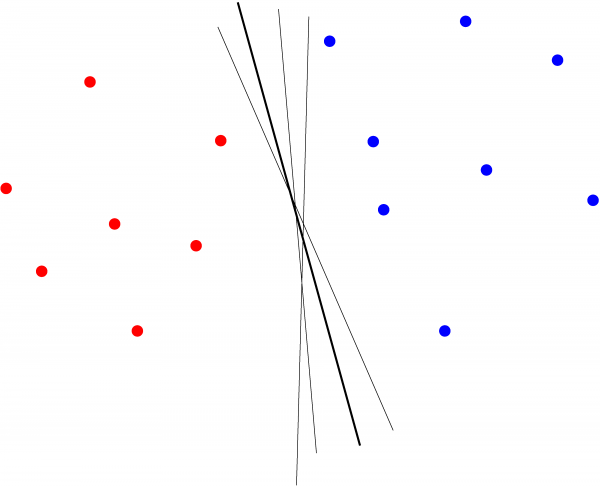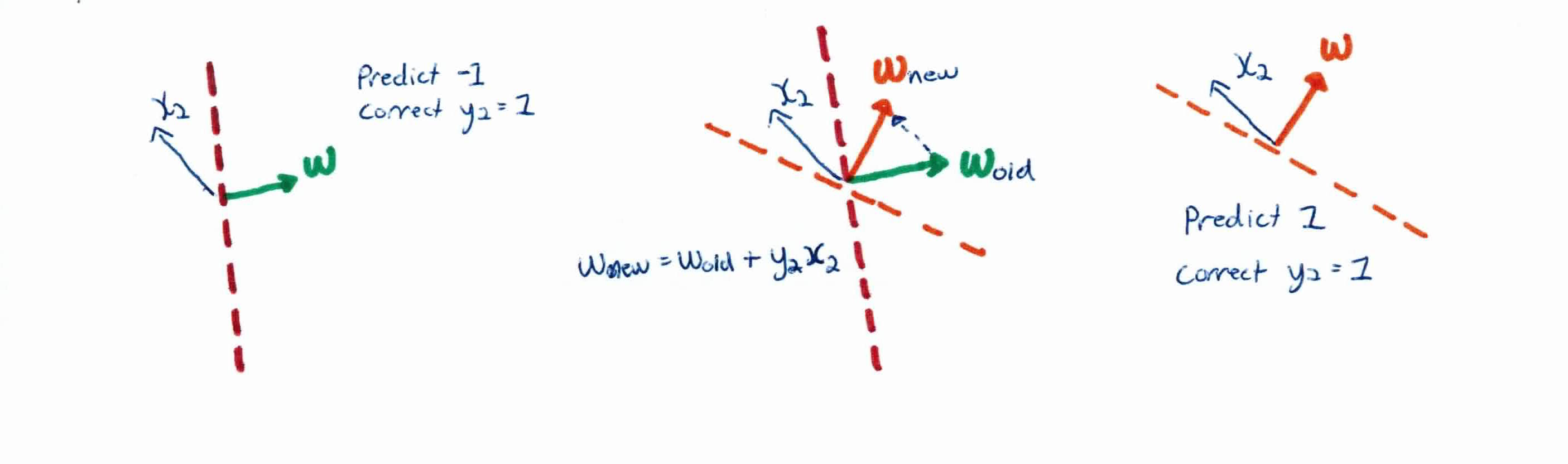# Abstract

The perceptron is a supervised learning algorithm that only works on linearly separable data, as its goal is to find a hyperplane to separate different classes. It is known as the single-layer neural network and is the most basic version of an artificial neuron. The perceptron takes in inputs with weights and runs it though a step-up function (instead of sigmoid like logistic regression) to see whether it should fire or not (return 1 or 0). Whenever the perceptron makes a mistake, the weights are updated on the spot to correct the mistake, progressively updating its hyperplane until it converges (finds separating hyperplane).

# Once Upon A Time

You’re the 45th. You say you want to keep America safe and stop criminals. You think the best way to do that is to build a straight, linear wall to separate US citizens and non-citizens (labels).

Your job is to find where you should build the wall. So, you have your best Immigration and Customs Enforcement (ICE) agents help you detect where to build the wall. You go to each person and have your ICE agents check whether they are here legally. They check all of their features like their green cards, SSN, visa, etc.

It they are above a threshold set by ICE, they will be considered illegal. If they are illegal and on the wrong side of the wall, you then move the (imaginary) wall, not the person, to have them separated. Once you have checked everyone in the United States and have found a linear wall separating documented and undocumented, you have your unpaid construction workers build the wall.

Any new person appearing on the other side of the wall is predicted to be undocumented. This, is in essence, how the perceptron works…

But, in reality, like many datasets, US citizens and non-citizens are not linearly separable. They are engrained into the communities and are all apart of the same dataset. Attempting to build a wall/hyperplane despite these facts will result in a loss. Accuracy will suffer, much like the 45th’s presidency. A better, more sophisticated plan/algorithm can be seen here.

# Perceptron

Recall that most algorithms model situations by creating a feature space, which is a finite-dimensional vector space, where each dimension represents a feature of your training examples.

For example, if you wanted to classify whether someone was an adult or child (labels), and you were given 2 features (height and weight), you would model it with a 2 dimensional graph.

In order to classify, you want to find a a hyperplane that will separate the data. So, when you get new data you want to classify, you just simply see what side of the hyperplane that new piece of data falls on.

The perceptron is a single layer feed-foward neural network with a simple output function. It uses a step-up, sign function and updates after every mistake. It looks for a separating line, but the line might not be optimal.These are some of the separating hyperplanes that perceptron can find. None are unique.

The perceptron is a linear method because the classification model is represented by a linear function $f$ (linear hyperplane) and is one of the simplest classification methods. It’s model is a basic neural network and only works for perfectly separated data. If data is not perfectly linear separable then the algorithm won’t converge. It can be used to represent many boolean functions: AND, OR, NAND, NOR, NOT, but not all of them (e.g., XOR).

The algorithm is given training data: $(x_1, y_1), \dots, (x_n, y_n)$ with $d$ features and it’s task is to learn a classification function $f: \mathbb{R}^d \rightarrow \mathbb{Y}$

The perceptron takes a linear combination of the weights (parameters) and features of an example and feeds it through a step function. If the linear combination exceeds the threshold, it fires, else, it doesn’t. This is similar to how the neurons in our brains fire. Our perceptron function is given as:

which can also be written as:

The weights (parameters) determine what the algorithm has learned. $w_i$ determines the contribution of $x_i$ to the label. $-w_0$ is a quantity that $\sum_{i=1}^n w_i x_1$ needs to exceed for the perceptron to output 1. The bias $w_0$ is also proportional to the offset of the plane from the origin. Recall that the weights $w_i$ determine the slope of the line and the weight vector is perpendicular to the plane.

Remember that our function $f(x_i)=sign(w_0 + \sum_{j=1}^d w_j x_{ij} )$ can be written as $f(x_i) = sign(\sum_{j=0}^d w_j x_{ij})$ if we set $x_{i,0}$ to 1.

How does the algorithm learn the weights? The idea is that it starts with a random hyperplane and it adjusts it using your training data. Every time the perceptron misclassifies a piece of data, $y_i f(x_i) \leq 0$, it adjusts it’s weight using the formula:Illustration of weights updating with $$\eta = 1$$

The algorithm converges once all data has been classified correctly.

There is no unique answer because you randomly initialize the weights and could end up with a different hyperplane every time you run perceptron.

The outcome is a perceptron defined by $(w_0, w_1, \dots, w_d)$.

# Algorithm

Given a training set ${(x_1, y_1), \ldots (x_n, y_n)}$, and learning rate $\eta$ the Perceptron algorithm works like this:

• Initialize the weights $w_j$ to $0$ for all $1 \leq j \leq d$.
• Repeat until convergence:
• For each example $x_i$ for all $1 \leq i \leq n$:
• If $y_i f(x_i) \leq 0$: //missclassification
• Update all $w_j$ with $w_j := w_j + \eta y_i x_i$ //adjust the weights

# Example

In our current training data, we have 2 undocumented immigrants with label 1 and 3 US citizens with label -1.

$$x_1 = (1,1), \ y_1 =$$1
$$x_2 = (2,0), \ y_2 =$$1
$$x_3 = (2,-2), \ y_3 =$$-1
$$x_4 = (-1,-2), \ y_4 =$$-1
$$x_5 = (-2,-1), \ y_4 =$$-1

We set our learning rate to $\eta=0.3$ and have our hyperplane pass through the origin by setting $w_0 = 0$ and $x_0 =0$. Randomizing our initial weights, we have them at:

So, writing out our decision boundary (wall):

We then follow our algorithm and go through each training example, attempting to classify them. If correct, we leave our wall alone. If incorrect, we update our weights, effectively moving our wall. We continue this process until convergence, i.e. all training examples are classified correctly.

Running the first example with our current weights, we get:

The above is not true, which means it was classified correctly. We get the same result with $x_2$. We run into our first misclassification with $x_3$:

This is an incorrect classification so we must use the update rule:

So we have:

Which represents our new wall.

Running through all of the examples we see that every example is now classified correctly. In practice, you will need to run through every example, multiple times, constantly updating all of the weights. We have built the wall, but we see that in real life, this is simply not efficient. Neural Networks are!

# Summary/Discussion

Perceptron is a supervised learning algorithm of binary classifiers, which are functions that decide whether an input belongs to some specific class or not. In the context of neural networks, a perceptron is an artificial neuron using the heaviside step function as the activation function and is the simplest feedforward neural network.

The perception takes an input with connecting weights $w$ and feeds it though a step function. If it passes the threshold, it fires and returns a 1. If not, it returns a 0. We want to train the perceptron to classify inputs correctly which is accomplished by adjusting the connecting weights. It does this by going through each example and adjusting it’s weight every time it makes a mistake. Consequently, it will never converge if the data is not linearly separable.

Other observations:

• The algorithm allows for online learning, in that it processes elements in the training set one at a time. It is also error-driven, whereas the parameters for logistic regression could be learned using a variety of batch algorithms, or an online algorithm, like stochastic gradient descent.
• Logistic regression returns a principled classification probability whereas perceptrons classify with a hard boundary.
• Logistic regression is a Generalized Linear Model (GLM) which can perform prediction and inference, whereas the linear Perceptron can only achieve prediction (in which case it will perform the same as logistic regression). The difference between the two is the fundamental difference between statistical modelling and machine learning.
...